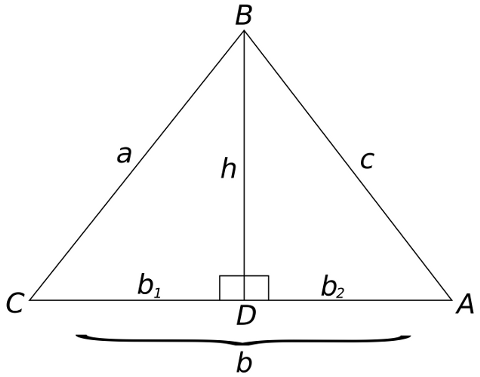#Heron's Formula

Math Lair Home > Topics > Heron's Formula

Heron's formula is a formula that relates the area of any triangle to the lengths of its sides. It is:

A = √s(sa)(sb)(sc)

In the above equation, a, b, and c are the lengths of the sides of the triangle, and s = ½(a + b + c); i.e. half of the perimeter of the triangle (also called the semiperimeter).

Heron's formula is usually proven geometrically or trigonometrically. An algebraic proof is presented below. If you'd like to skip the proof and continue to further discussion, select this link.Consider triangle ABC, having side lengths a, b, and c. Draw a line through B perpendicular to CA, meeting CA at D. Call its length h, and call the lengths of CD and DA b1 and b2, respectively (note that one of b1 and b2 may be negative if the triangle is obtuse). This is all shown in the diagram above.

First, express b1 and b2 in terms of a, b, c, and h, then express h in terms of a, b, and c, and then evaluate Area = ½bh:

Using the Pythagorean theorem:

h² + b2² = c²
b2 = √c² − h²
As well:
b1 = bb2
b1 = b − √c² − h²

Now, express h in terms of a, b, and c:

h² + b1² = a²
h² = a² − (b − √c² − h²
h² = a² − (b² − 2bc² − h² + c² − h²)
h² = a² − b² + 2bc² − h²c² + h²
b² + c² − a²2b = √c² − h²
h² = c² − b² + c² − a²2b
h = √(c + b² + c² − a²2b)(cb² + c² − a²2b)

Having expressed h in terms of the sides of the triangle,

Area = ½bh = ¼√(4b²c² −(b² + c² − a²)²)
= ¼√(2bc + b² + c² − a²)(2bcb² − c² + a²)
= ¼√((b + c)² − a²)(a² − (bc)²)
= ¼√(b + ca)(b + c + a)(a + bc)(ab + c) = √½(a + b + c)·½(a + b + c − 2a)·½(a + b + c − 2b)·½(a + b + c − 2c)
Area= √s(sa)(sb)(sc)

It is possible to compute the area of a triangle when given the lengths of its sides. Is it possible to compute the area of a quadrilateral given its side lengths? The answer is no; two quadrilaterals whose sides are all the same could have different lengths. Unlike triangles, quadrilaterals are not rigid. You can demonstrate this for yourself. Take three straws (or other straight objects) and use balls of clay or large marshmallows (or something similar) to attach them in the shape of a triangle. Now try moving one of the straws without detaching it from the two balls of clay it's attached to. You won't be able to do it; the shape is rigid. Now create a square. This time, the square will readily deform into a parallelogram.

However, it can be shown that the maximum area of a quadrilateral with sides a, b, c, and d is:

A = √(sa)(sb)(sc)(sd)